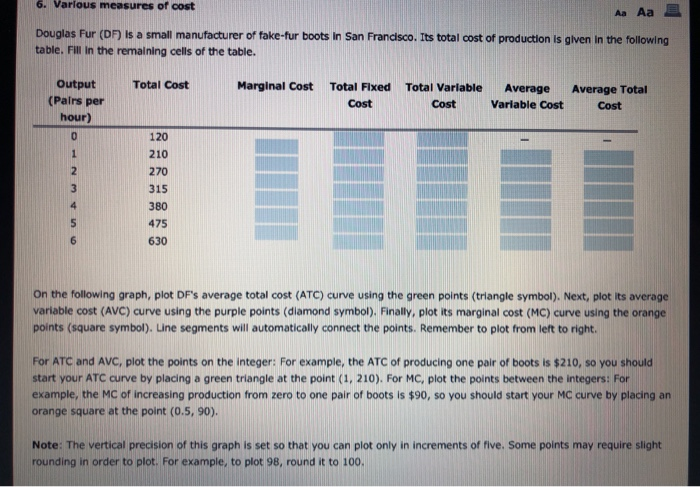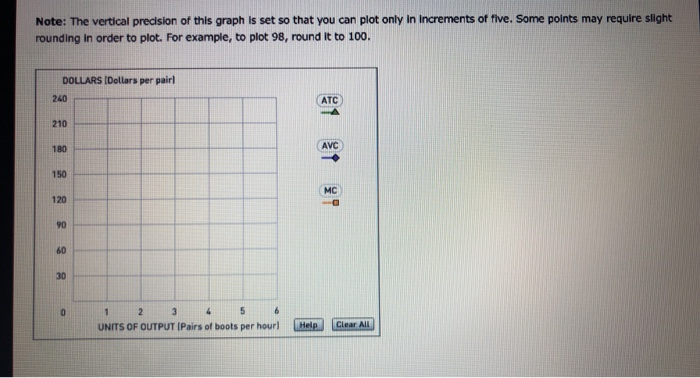### Create an Account

Home / Questions / Please indicate the specific coordinate points and distinguish them by different colors. Thank you f

# Please indicate the specific coordinate points and distinguish them by different colors. Thank you f

Please indicate the specific coordinate points and distinguish them by different colors. Thank you for your help and work!6. Various measures of cost А. Аа Douglas Fur (DF) is a small manufacturer of fake fur boots in San Francisco. Its total cost of production is given in the following table. Fill in the remaining cells of the table. Total Cost Marginal Cost Output (Pairs per hour) Total Fixed Cost Total Variable Cost Average Variable Cost Average Total Cost ع م به لا ط م و 630 On the following graph, plot DF&#39;s average total cost (ATC) curve using the green points (triangle symbol). Next, plot its average variable cost (AVC) curve using the purple points (diamond symbol). Finally, plot its marginal cost (MC) curve using the orange points (square symbol). Une segments will automatically connect the points. Remember to plot from left to right. For ATC and AVC, plot the points on the integer: For example, the ATC of producing one pair of boots is \$210, so you should start your ATC curve by placing a green triangle at the point (1, 210). For MC, plot the points between the integers: For example, the MC of increasing production from zero to one pair of boots is \$90, so you should start your MC curve by placing an orange square at the point (0.5, 90). Note: The vertical precision of this graph is set so that you can plot only in increments of five. Some points may require slight rounding in order to plot. For example, to plot 98, round it to 100. Note: The vertical precision of this graph is set so that you can plot only in increments of five. Some points may require slight rounding in order to plot. For example, to plot 98, round it to 100. DOLLARS (Dollars per pair 240 UNITS OF OUTPUT IPairs of boots per hourl Help Clear All

Apr 03 2020 View more View LessSubscribe To Get Solution